# Pipeline Structural Comparison BUT

## ST Comparison BUT

Description:

This is the Graphical User Interface (GUI) tutorial for the pipeline that compares two groups of subjects using structural data (such as MRI) and binary undirected graphs at fixed thresholds.

In this tutorial, we will use the example data, but you can use your own data as well (for instructions on how to prepare your own functional data to be analyzed in BRAPH2, see tutorial Structural Data format).

• Start BRAPH 2 and select the Pipeline from the main GUI

Start MATLAB, navigate to the BRAPH2 folder and run “braph2” with the following command:

>> braph2

Select the Pipeline Structural Comparison BUT in the right menu. You can use the search field, typing structural for example (Figure 1).Figure 1. Landing GUI.

Once you click on the pipeline, the window in Figure 2 will open.Figure 2. Landing pipeline GUI

The first step is to load a brain atlas from an XLS file. Press the button “Load a Brain Atlas XLS” (the only one active in the pipeline GUI, Figure 2) and then select the destrieux_atlas.xlsx in the directory ./braph2/pipelines/structural/example data ST (MRI) (Figure 3).Finally, to visualize the atlas press “Plot the brain atlas” and play with the surface settings (Figure 4). Check the Brain Atlas for more information on how to control the appearance of this interface.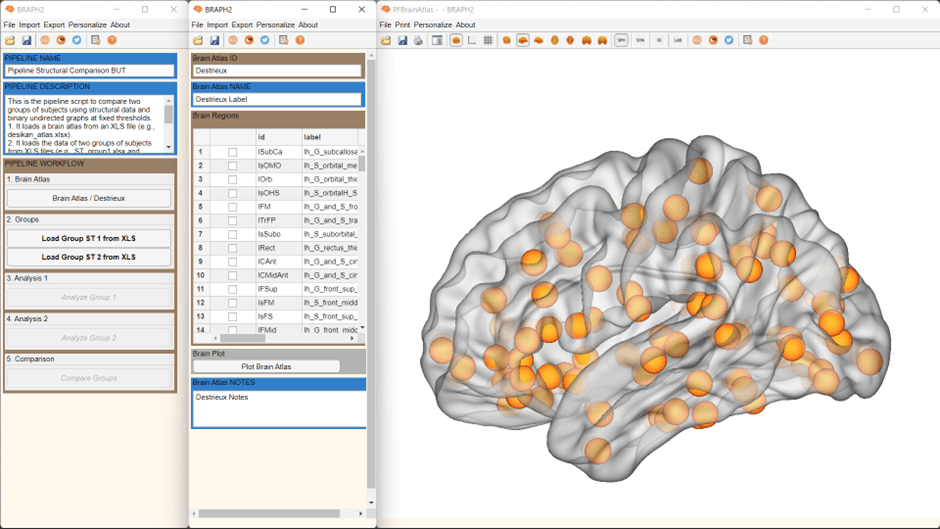Figure 4. Plot the brain atlas.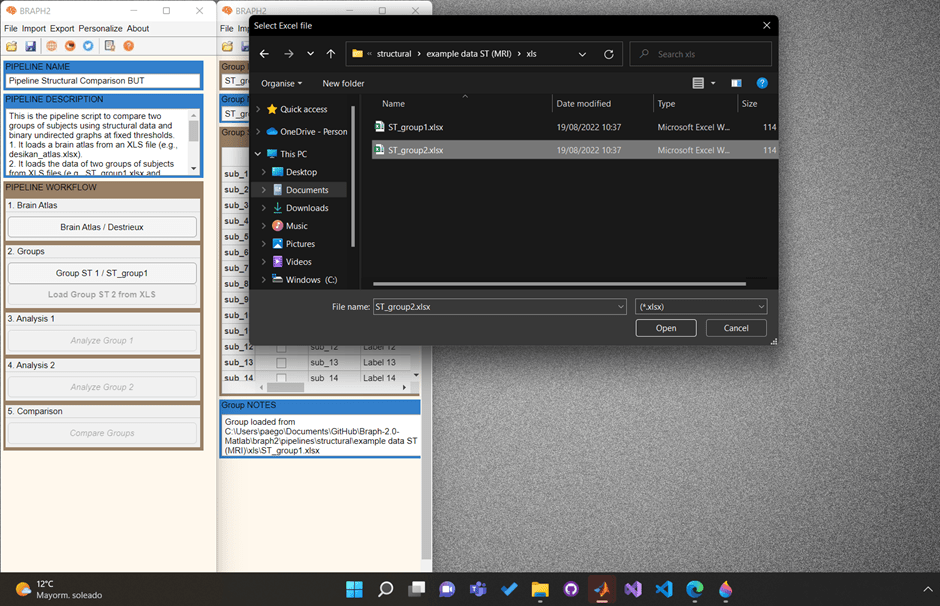Figure 5. From the pipeline GUI, first load data for group 1 (ST_group1.xlsx) and then for group 2 (ST_group1.xlsx).

To load the data for the two groups you would like to compare press “Load Group ST 1 from XLS” and select the file for subjects of Group1 which is at the directory ./braph2/pipelines/structural/example data ST (MRI)/xls/ST_group1. After, load the data for the group 2 which can be found at directory ./braph2/pipelines/structural/example data ST (MRI)/xls/ST_group2 (Figure 5).

• Performing the structural analysis of group 1

Analysis of the first group using structural analyses (ST) based on binary undirected graphs at fixed thresholds (BUT).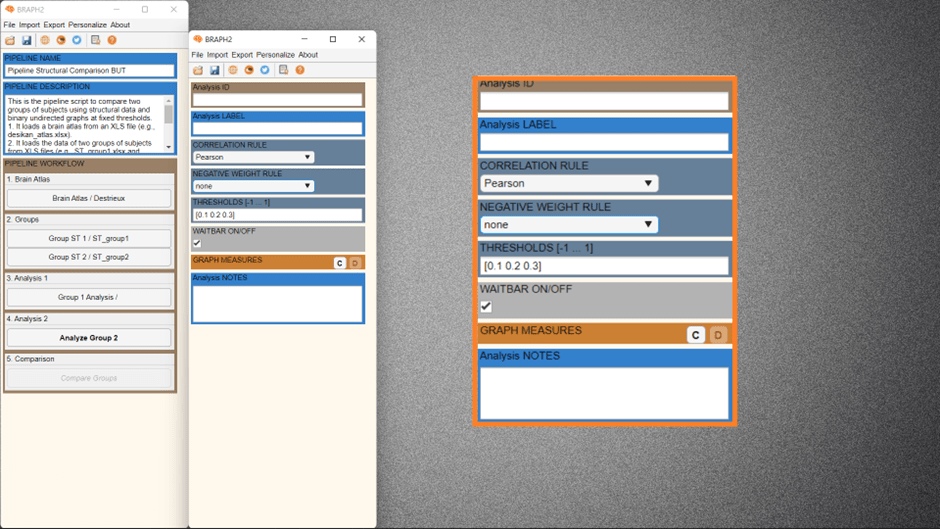Figure 6. After pressing Analyze group 1, the interface for Analysis of group 1 opens.

First press “Analyze Group 1” to open the interface for its analysis (Figure 6). In this interface, you can specify the parameters for constructing the graph from the MRI group data. Especially, in the thresholds section, you can specify the thresholds by filling “[0.1 0.2 0.3]” or “0.1:0.1:0.3”. To plot the graph for the whole group go to the section GRAPH MEASURES and press “C”, right click on the left of a measure to deploy the menu seen on Figure 7 and select “Plot Graph”.Figure 7. Plot graph. By default, we see the adjacency matrix from the first threshold.

Press the settings panel button in Figure 8, a settings panel will show up. In the last section “DT” of this panel, we can select at which threshold to show the adjacency matrix.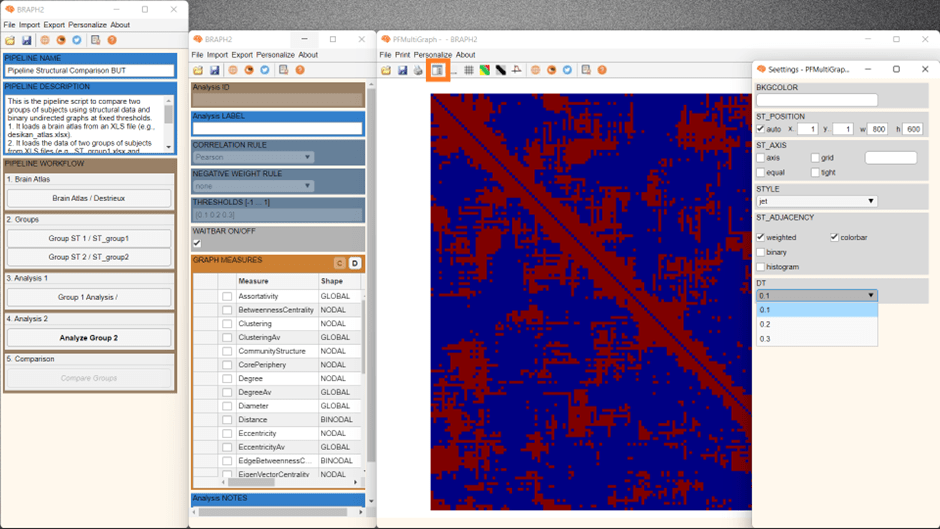Figure 8. Plot graph at a fixed threshold.

Now it is the moment to calculate some connectivity measures for group 1. Select one measure, for example, Degree (nodal), and calculate by right clicking on the left-side of the measures list and press “Calculate Selected Measures”. When the measure is calculated, a C appears on its left, and now, again by right clicking on the left-side of the measures list and pressing “Plot Selected Measures Brain Graph” (see Figure 9), we will be able to visualize the results of Degree for group 1.Figure 9. When the measure is calculated, the C appears and then you can plot it on a brain surface.

A new figure with the brain surface will open with default settings for brain region visualization. If we go down in the Settings figure, we will find Measures, DT and EDGES panels. By checking Measures box, we can adapt the size and the color of the brain regions spheres to be proportional to the measure’s value (Figure 10), where blueish colors represent smaller values, and red larger.  In DT panel we changed the threshold from 0.1 to 0.3, and then we selected the checkbox in EDGES panel to see the edges at that threshold (Figure 10).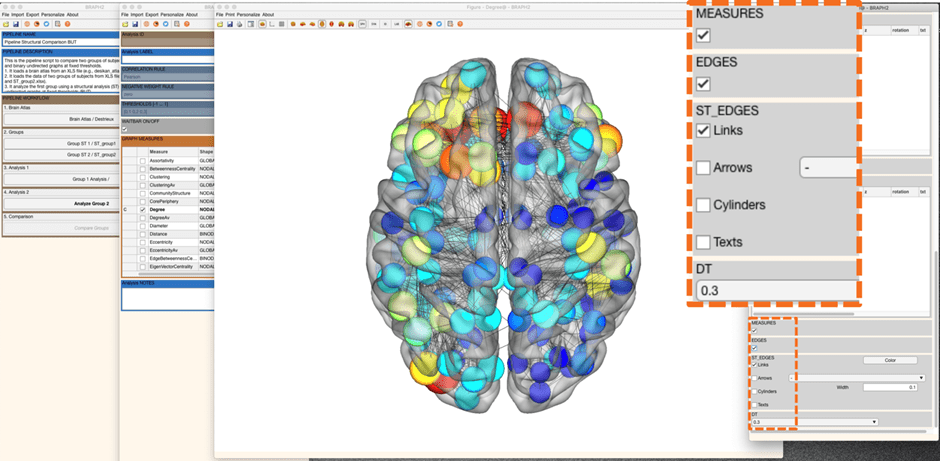Figure 10. Show the data of the selected measure.

We can also first press “Data Selected Measures” from the GRAPH MEASURES menu mentioned earlier, and then a figure will open showing the array of values for the measure (Figure 11), and from there we can press “Plot Brain Multi Graph” to visualize the results of Degree for group 1 on a brain surface, and obtain the same plot as in Figure 10.

In case we want to calculate a measure with different rules or parameters, it is done by going first to “Data Selected Measuresbefore calculating the measure. For more details, check the section Measures’ calculation with different parameters.Figure 11. Show the data of the selected measure.

• Performing the structural analysis of group 2

Analysis of the second group using the same parameters selected for the first group (structural analyses (ST) based on binarized undirected graphs at fixed thresholds (BUT)).

Follow the previous steps in the analysis of group 1 and explore some other measures for group 2.

• Comparing the two groups

To proceed with the group comparison press “Compare Groups” and the interface to select the comparison parameters will open (Figure 12). After setting the desired permutation number (recommended 1000 – 10000 permutations), press “C” under the GROUP COMPARISONS section to open the list of measures to use to compare the groups.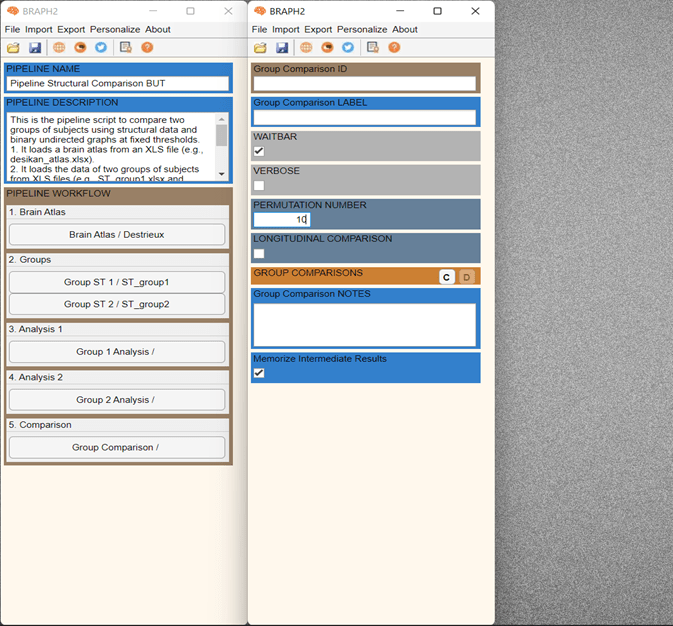Figure 12. Open the Group Comparison interface.

It is possible to select multiple measures to be compared, and then right-click on the left of the measure table and select “Calculate Selected Comparisons” (Figure 13). Depending on how many permutations you use it will take less or more time.

Once the calculations are done, you should see a C on the left of the measure to be compared (Figure 14). Then press “Data Selected Comparison” to show the different value between groups.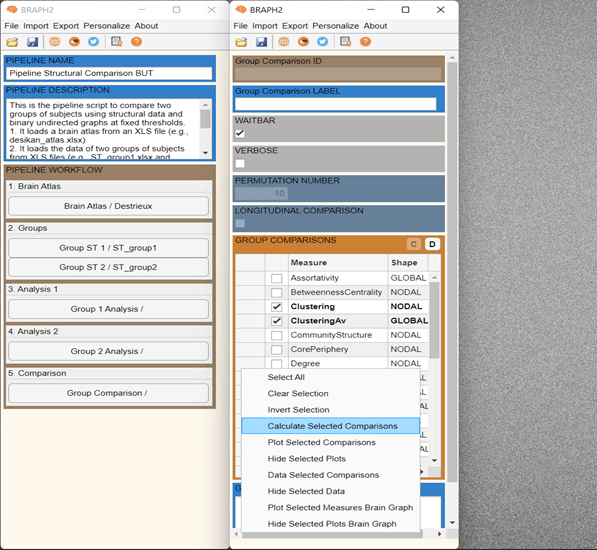Figure 13. Calculation of the comparison.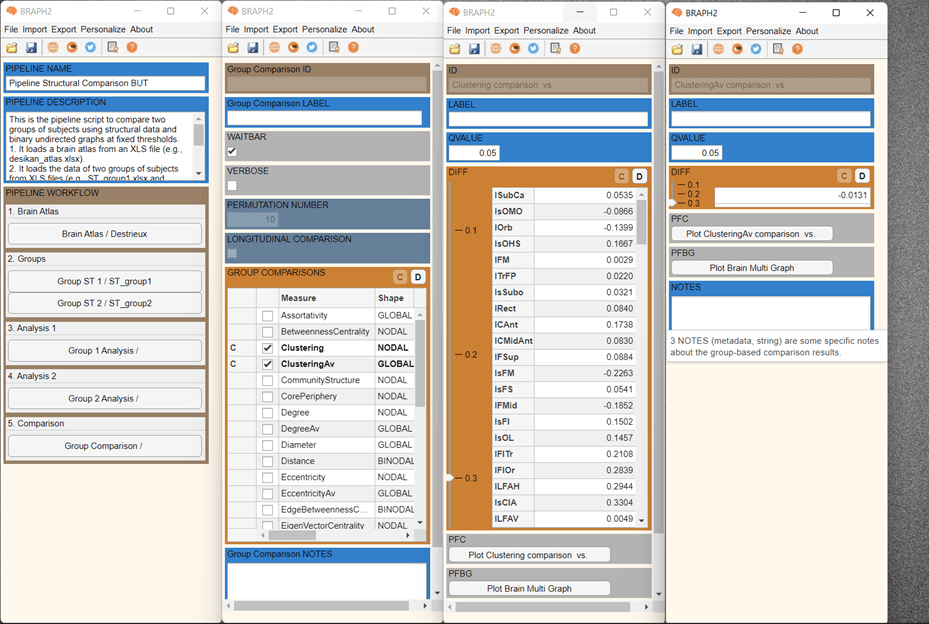Figure 14. Show the results of the comparisons of multiple measures.

We can also plot the results from the comparison by pressing “Plot clustering Comparison” for the global measures and “Plot Brain Multi Graph” for the nodal measures. If we check the “Plot Brain Multi Graph”, we can see the differences between groups and if they are significant. If we go down in the Settings figure, we will find MEASURES, FDR SHOW, QVAL, and DT panels. By checking the Measures box and changing the threshold to one threshold of interest, for example 0.1 we can see differences in both directions. Differences in red mean that clustering is larger in group 2 than in group 1, whereas differences in blue means that clustering is larger in group 1 than in group 2.Figure 15. Differences in clustering between group 1 and group 2.

By checking the FDRSHOW box, just the regions that are significantly different between groups after applying FDR correction at the desired level (in the Figure 16, q value is set at 0.05) will be shown, and the rest will be hidden. In this case any region survives after FDR correction.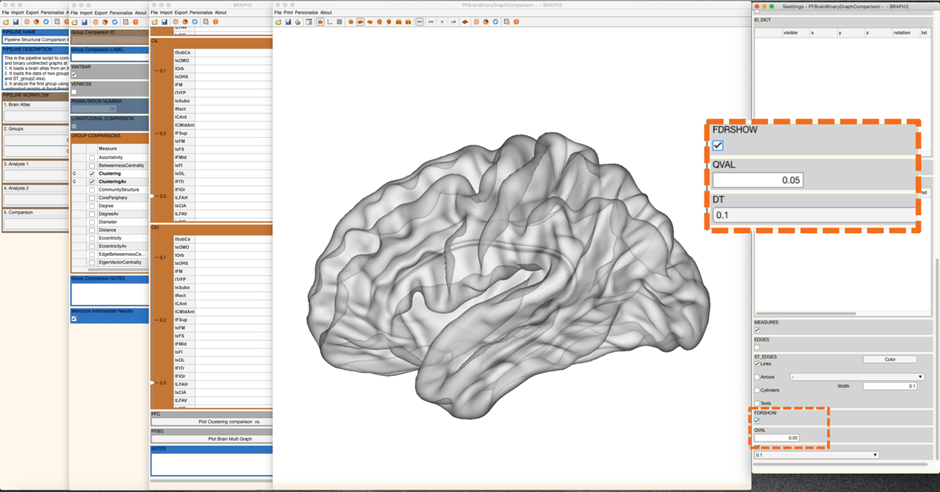Figure 16. No regions are significant after applying FDR at a q_value = 0.05.

We can also plot the results of global measure (in this case, ClusteringAv) from the comparison by pressing “Plot ClusteringAv comparison vs.” in the measure’s table GUI (Figure 17). In this plot we can see the differences in global clustering values between the groups at each density (Orange points). The lines from the black points indicate the upper and lower confidence intervals. In the settings panel, we can change the text of the x axis and the y axis.Figure 17. Plot of a global measure comparison.